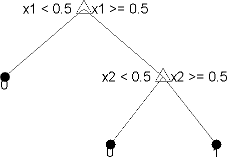Documentation

### This is machine translation

Mouseover text to see original. Click the button below to return to the English version of the page.

Note: This page has been translated by MathWorks. Click here to see
To view all translated materials including this page, select Country from the country navigator on the bottom of this page.

## Decision Trees

Decision trees, or classification trees and regression trees, predict responses to data. To predict a response, follow the decisions in the tree from the root (beginning) node down to a leaf node. The leaf node contains the response. Classification trees give responses that are nominal, such as `'true'` or `'false'`. Regression trees give numeric responses.

Statistics and Machine Learning Toolbox™ trees are binary. Each step in a prediction involves checking the value of one predictor (variable). For example, here is a simple classification tree:This tree predicts classifications based on two predictors, `x1` and `x2`. To predict, start at the top node, represented by a triangle (Δ). The first decision is whether `x1` is smaller than `0.5`. If so, follow the left branch, and see that the tree classifies the data as type `0`.

If, however, `x1` exceeds `0.5`, then follow the right branch to the lower-right triangle node. Here the tree asks if `x2` is smaller than `0.5`. If so, then follow the left branch to see that the tree classifies the data as type `0`. If not, then follow the right branch to see that the tree classifies the data as type `1`.

To learn how to prepare your data for classification or regression using decision trees, see Steps in Supervised Learning.

### Train Classification Tree

This example shows how to train a classification tree.

Create a classification tree using the entire `ionosphere` data set.

```load ionosphere % Contains X and Y variables Mdl = fitctree(X,Y)```
```Mdl = ClassificationTree ResponseName: 'Y' CategoricalPredictors: [] ClassNames: {'b' 'g'} ScoreTransform: 'none' NumObservations: 351 Properties, Methods ```

### Train Regression Tree

This example shows how to train a regression tree.

Create a regression tree using all observation in the `carsmall` data set. Consider the `Horsepower` and `Weight` vectors as predictor variables, and the `MPG` vector as the response.

```load carsmall % Contains Horsepower, Weight, MPG X = [Horsepower Weight]; Mdl = fitrtree(X,MPG)```
```Mdl = RegressionTree ResponseName: 'Y' CategoricalPredictors: [] ResponseTransform: 'none' NumObservations: 94 Properties, Methods ```

 Breiman, L., J. H. Friedman, R. A. Olshen, and C. J. Stone. Classification and Regression Trees. Boca Raton, FL: Chapman & Hall, 1984.

Download ebook